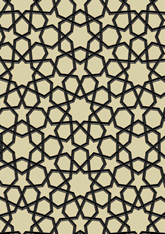# Qaytbay mosquedata188/FIG2

## Geometry

• The symmetry group of the tiling is *632 (p6m).
• All the internal angles of the constituent polygons are a multiple of 2°.
• Contains one regular 6-pointed star polygon with vertex angle of 76°.
• Contains one regular 9-pointed star polygon with vertex angle of 92°.
• There are 7 non-regular reflective tiles (including one kite).
• The tiling satisfies the interlace condition and has one finite interlace and two infinite interlaces with straight cross-overs.
• The tiling is edge-to-edge.
• As drawn, contains about 348 polygons.

## References

Publications referenced: